# coplanarWaveguide

Create coplanar waveguide transmission line

Since R2021b

## Description

Use the `coplanarWaveguide` object to create a coplanar waveguide transmission line. A Coplanar waveguide is a common type of transmission line used in any PCB implementation of RF and microwave components. A coplanar waveguide transmission line has a center conductor strip and two ground planes. One ground plane is the layer that acts as the conductor strip and the other ground plane is the bottom layer.

Note

This PCB object supports behavioral modeling. For more information, see Behavioral Models. To analyze the behavioral model for a coplanar waveguide, set the `Behavioral` property in the `sparameters` function to `true or 1`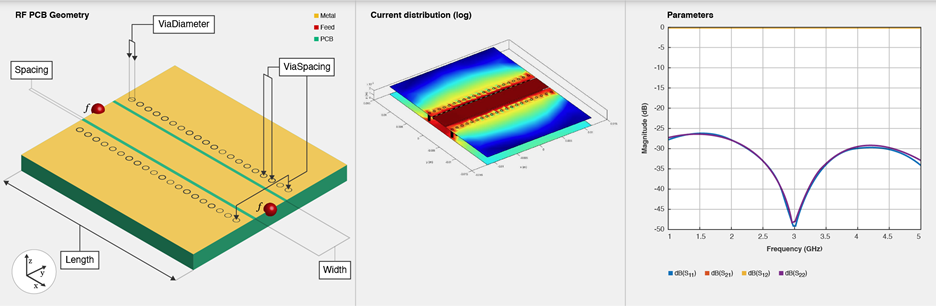## Creation

### Syntax

``cpgw = coplanarWaveguide``
``cpgw = coplanarWaveguide(Name=Value)``

### Description

example

````cpgw = coplanarWaveguide` creates a default coplanar waveguide transmission line with a Teflon substrate and default property values for a 50 ohm transmission line.```
````cpgw = coplanarWaveguide(Name=Value)` sets Properties using one or more name-value arguments. For example, `coplanarWaveguide(Width=0.0047)` creates a coplanar waveguide transmission line of width 0.0047 meters. Properties not specified retain their default values.```

## Properties

expand all

Length of the coplanar waveguide transmission line in meters, specified as a positive scalar.

Example: `cpgw = coplanarWaveguide(Length=0.0300)`

Data Types: `double`

Width of the coplanar waveguide transmission line in meters, specified as a positive scalar.

Example: `cpgw = coplanarWaveguide(Width=0.0047)`

Data Types: `double`

Distance between the transmission line and the adjacent top layer metal of the ground plane, specified as a positive scalar in meters.

Example: `cpgw = coplanarWaveguide(Spacing=3.0000e-04)`

Data Types: `double`

Distance between the vias in meters, specified as a two-element vector of positive elements.

Example: `cpgw = coplanarWaveguide(ViaSpacing=[0.0021 0.0060])`

Data Types: `double`

Diameter of the via in meters, specified as a positive scalar.

Example: `cpgw = coplanarWaveguide(ViaDiameter=7.0000e-04)`

Data Types: `double`

Height of the coplanar waveguide transmission line from the ground plane, specified as a positive scalar in meters.

Example: `cpgw = coplanarWaveguide(Height=0.0020)`

Data Types: `double`

Width of the ground plane in meters, specified as a positive scalar.

Example: `cpgw = coplanarWaveguide(GroundPlaneWidth=0.0350)`

Data Types: `double`

Type of dielectric material used as a substrate, specified as a `dielectric` object. The dielectric material in a `coplanarWaveguide` object with default properties is Teflon.

Example: `d = dielectric("FR4");` `cpgw = coplanarWaveguide(Substrate=d)`

Data Types: `string` | `char`

Type of metal used in the conducting layers, specified as a `metal` object. The type of metal in a `coplanarWaveguide` object with default properties is PEC.

Example: `m = metal("Copper");` `cpgw =coplanarWaveguide(Conductor=m)`

Data Types: `string` | `char`

## Object Functions

 `charge` Calculate and plot charge distribution `current` Calculate and plot current distribution `design` Design coplanar waveguide transmission line around particular frequency `feedCurrent` Calculate current at feed port `getZ0` Calculate characteristic impedance of transmission line `mesh` Change and view mesh properties of metal or dielectric in PCB component `shapes` Extract all metal layer shapes of PCB component `show` Display PCB component structure or PCB shape `sparameters` Calculate S-parameters for RF PCB objects

## Examples

collapse all

Create a coplanar waveguide transmission line.

`waveguide = coplanarWaveguide`
```waveguide = coplanarWaveguide with properties: Length: 0.0231 Width: 0.0039 Spacing: 2.0000e-04 ViaSpacing: [0.0011 0.0070] ViaDiameter: 5.0000e-04 Height: 0.0016 GroundPlaneWidth: 0.0300 Substrate: [1x1 dielectric] Conductor: [1x1 metal] ```

View the coplanar waveguide transmission line.

`show(waveguide)`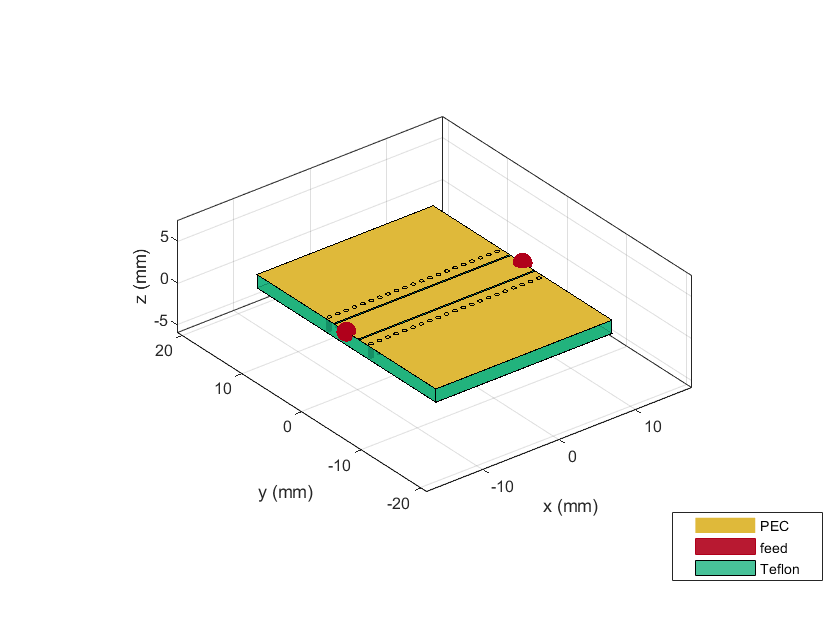Calculate the S-parameters of the waveguide from 1-10 GHz.

`sparam = sparameters(waveguide,1e9:0.3e9:10e9)`
```sparam = sparameters: S-parameters object NumPorts: 2 Frequencies: [31x1 double] Parameters: [2x2x31 double] Impedance: 50 rfparam(obj,i,j) returns S-parameter Sij ```

Plot the S-parameters.

`rfplot(sparam)`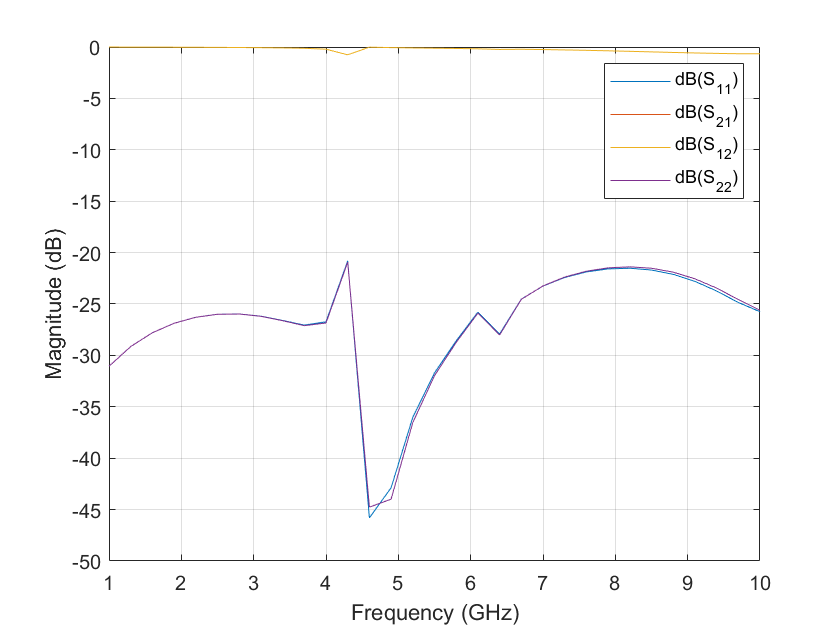Create a coplanar waveguide transmission line using a gold substrate as the dielectric.

```txem = coplanarWaveguide; txem.Conductor = metal("Gold");```

Design the coplanar waveguide at a frequency of 3 GHz, line length of 0.5 meters, and impedence of 75 ohms.

```txem = design(txem,3e9,LineLength=0.5,Z0=75); show(txem)```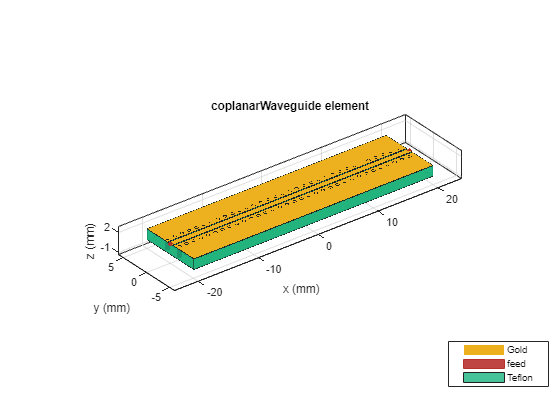Compute and plot the behavioral S-parameters of the waveguide.

```spar = sparameters(txem,3e9,Behavioral=true); rfplot(spar)```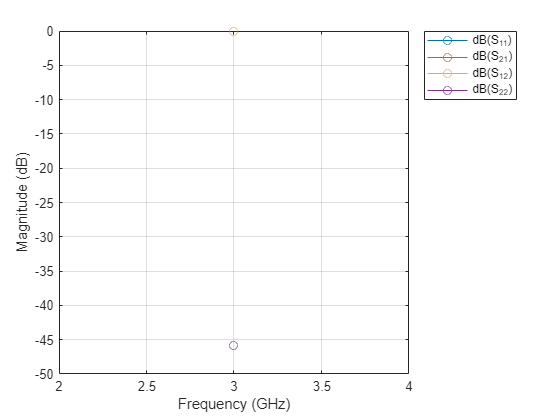Pozar, David M. Microwave Engineering. 4th ed. Hoboken, NJ: Wiley, 2012.